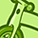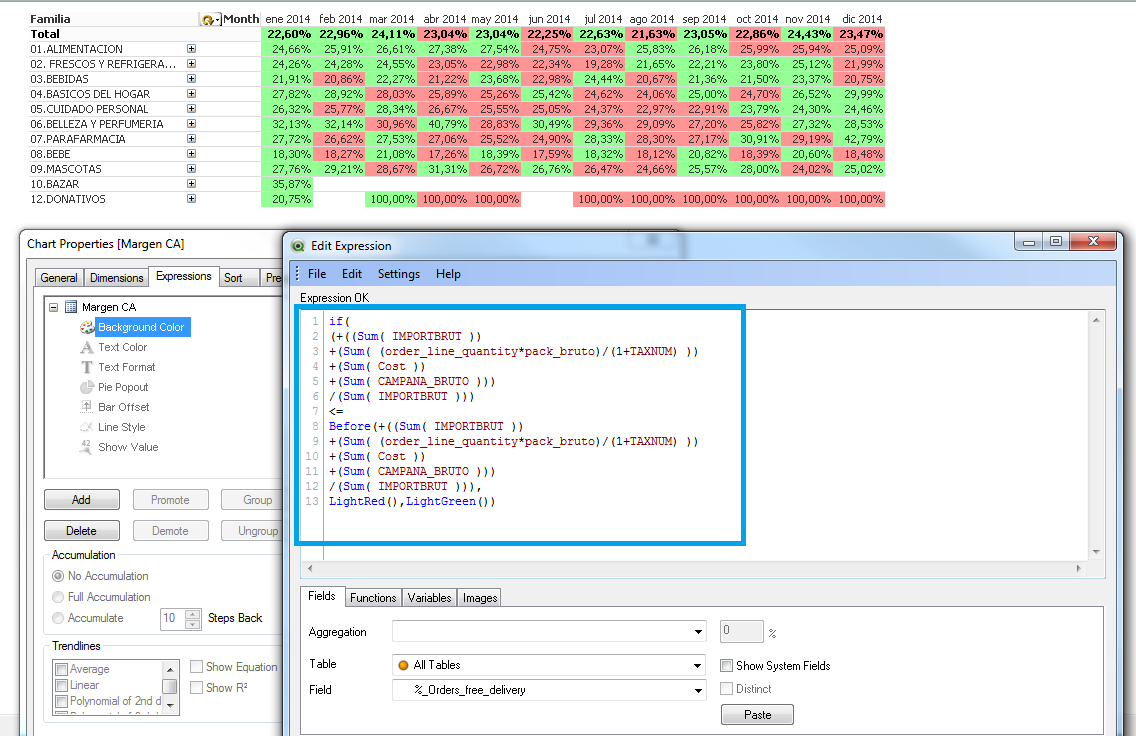# QlikView App Dev

Discussion Board for collaboration related to QlikView App Development.

cancel
Showing results for
Did you mean:Creator II

## Change color if the margin is better than preivous month

Hi all

I just building a table to show the margins of our categories. I want to show the variation from previous month, if the margin is lower than the previous month the cell is in red, if not, in green...

See the file attached to see the example that did not work

Many thank's

1 Solution

Accepted SolutionsMVP

Hi Eduard you can try to change your background color expression to:

If([Margen CA]<=before([Margen CA]),LightRed(),LightGreen())

4 RepliesMVP

Hi Eduard you can try to change your background color expression to:

If([Margen CA]<=before([Margen CA]),LightRed(),LightGreen())Creator III

Hi Eduard,

If you keep 'Month' field on horizontal you can use Before(), if you want to display months on vertical you can use Above():

if(

(+((Sum( IMPORTBRUT ))

+(Sum( (order_line_quantity*pack_bruto)/(1+TAXNUM) ))

+(Sum( Cost ))

+(Sum( CAMPANA_BRUTO )))

/(Sum( IMPORTBRUT )))

<=

Before(+((Sum( IMPORTBRUT ))

+(Sum( (order_line_quantity*pack_bruto)/(1+TAXNUM) ))

+(Sum( Cost ))

+(Sum( CAMPANA_BRUTO )))

/(Sum( IMPORTBRUT ))),

LightRed(),LightGreen())Best regards,

DavidMVP

Or, for get the color also in first month, use TOTAL in 2º part of the if sentence:

if(

(+((Sum( IMPORTBRUT ))

+(Sum( (order_line_quantity*pack_bruto)/(1+TAXNUM) ))

+(Sum( Cost ))

+(Sum( CAMPANA_BRUTO )))

/(Sum( IMPORTBRUT )))

<=

(+(SUM(TOTAL <DES_FAMILIA, DES_SUB1, DES_SUB2, ITEMID> {<CalendarMonthName={'\$(VPriorMonth)'},DATEFINANCIAL=>}IMPORTBRUT)

+(SUM(TOTAL <DES_FAMILIA, DES_SUB1, DES_SUB2, ITEMID> {<CalendarMonthName={'\$(VPriorMonth)'},DATEFINANCIAL=>}(order_line_quantity*pack_bruto)/(1+TAXNUM)))

+SUM(TOTAL <DES_FAMILIA, DES_SUB1, DES_SUB2, ITEMID> {<CalendarMonthName={'\$(VPriorMonth)'},DATEFINANCIAL=>}Cost)

+SUM(TOTAL <DES_FAMILIA, DES_SUB1, DES_SUB2, ITEMID> {<CalendarMonthName={'\$(VPriorMonth)'},DATEFINANCIAL=>}CAMPANA_BRUTO))

/SUM(TOTAL <DES_FAMILIA, DES_SUB1, DES_SUB2, ITEMID> {<CalendarMonthName={'\$(VPriorMonth)'},DATEFINANCIAL=>}IMPORTBRUT)),

LightRed(),LightGreen())Creator II
Author

Hi Ruben

Many many thank's

Problem fixed 🙂

Eduard